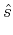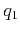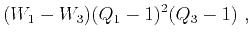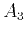On anelliptic approximations for qP velocities in TI and orthorhombic mediaNext: Moveout approximation Up: Transversely isotropic media Previous: Previous Approximations

## Proposed Approximations

To derive a more symmetric form, we return to the four-parameter expressions (equations 17 and 18) and propose to modify them as follows:(23)

and(24)

where(25)(26)

The modifications in equation 25 are equivalent to the second anelliptic approximations by Dellinger et al. (1993). Again, parametersandcan be found by fitting the velocity profile curvatures at the vertical () and horizontal () axis, respectively and are defined in equations 1 and 2. Analogously,andcan be found by fitting the fourth-order derivative () at the same angle. A similar strategy applies to fitting parameters for the group-velocity approximation. Note that expressions forandare different from equations 19 and 20.

Following this approach, we derive the following expressions for,,, and:(27)(28)(29)(30)Note that equations 23 and 24 introduce three more parameters generating six parameters in total, namely,,,,, andfor equation 23 or,,,,, andfor equation 24. However, expressingandin terms ofandin equations 27-30, we effectively reduce the dependency to four parameters. This reduction leads to four-parameter anelliptic approximations, which fit up to the fourth-order accuracy along both axes. The exact phase- and group-velocity expressions also require the total of four independent parameters. However, the advantage of the proposed approximations lies in the existence of the group-velocity expression (equation 24) with analogous functional form as the phase-velocity expression (equation 23). To reduce the number of parameters to three, we utilize the linear relationships betweenandgiven in Figure 1. The requiredandparameters for the group-velocity approximations can be found from the reciprocals ofandfor phase-velocity approximations, as mentioned above. Therefore, both phase- and group-velocity approximations derived on the basis of this approach require the same number of parameters.

SubsectionsOn anelliptic approximations for qP velocities in TI and orthorhombic mediaNext: Moveout approximation Up: Transversely isotropic media Previous: Previous Approximations

2017-04-14